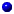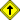Introduction
Overview
History
Books
Tutorials

3D on the Web
Pure Java
Java3D
Flash
VRML
Other

Modeling
The idea of modelling not only contains the format of the resulting model, but the way in which the model is generated. Considerable development is ongoing in both areas.OverviewPolygon DefinitionOther TechniquesReturn to top of document

### Modelling Overview

In an earlier part of this site I wrote:
"The first step of creating photo-realisitc 3D images is to create a polygonal model of the objects in the scene"
This is a very broad brush of a what can be a complicated task. What actually happens first is that a mathematical model of the object is created. Then, the surface of the model is fitted with polygons to which one of the many rendering techniques may be applied. (Note: as I've mentioned there are rendering techniques which do not require the use of polygons, so this approach is not applicable in every case)

Even the phrase "mathematical model" is not exactly an accurate description of what modelling is about. For example, the model of a simple cube could be a simple listing of 12 triangles (2 triangles for each of the 6 sides of a cube). The list of triangles completely describes the surface of the cube - no 'mathematical model' is required.

However, for more complicated objects 'mathematical model' may be exactly the right description. For example, to model a sphere you would use the equation:

r^2 = x^2 + y^2 + z^2
This describes the points (x,y,z) on a spherical surface with radius r and center (0,0,0).

In the 3D industry, simple objects such as spheres, cones, and cylinders are often used to create simple scenes because they can be easily modelled by equations.

For more complicated scenes, with multiple objects, combinations of techniques may be used to completed describe all of the objects in a scene.

In most real-world scenes the objects are very complex and it is rare that a simple listing of polygons or a single equation is all the modelling that is needed.

Consider, for example, a human face. No single equation can describe the various surface curvatures and extensions (ears, hair) that make up a face.

Much of the work in the 3D graphics industry is about devising mathematical techniques for describing surfaces. No one approach has been developed which satisifies all modelling needs and 3D graphics designers continue to employ multiple techniques. There are primarily three types of mathematical surface descriptions used by 3D graphics designers, all of which are fairly complicated to program and which require significant computational power. These techniques are used because of the excellent results they provide, despite the difficulties in working with them.

• Bezier spline
• B-spline
• NURBSReturn to top of document

### Polygon Definition

As I've stated before, the rendering techniques used in the 3D industry are focussed on shading polygons. So once the model of a scene is completed, step number two is to define the polygons which make up the objects.

For reasons I'll go into shortly, there are constraints on the definition of the polygonal 'mesh' that must be observed. In particular, all polygon vertices must coincide with the vertices of other polygons. For example, the corners of all triangles must touch other triangles only at their corners. This constraint can also be stated that all vertices must be shared vertices. The index of one polygon must not touch the edge of another polygon in the "middle" (on the line segment between two vertices).

Any polygonal mesh which breaks these rules is no less an accurate model of the objects in a scene. However, the rendering techniques that we've describe earlier cannot be applied.

For example, Gouraud shading requires that the colorization of a vertex be defined as the average between the polygons which share that vertex. So if a vertex is shared by only one polygon, the Gouraud shading algorithm would not provide the smooth shading that is desired.

This same problem exists for essentially all of the shading techniques. Common vertices are needed to enable smooth shading algorithms to be effective.

In all the reading and work I've done on 3D graphics, the most under-discussed topic I've found is the technique for creating the polygons that make up the surface of an object. Perhaps I've missed the body of work which describes the techniques for covnerting models to vertices, but if so then it's well hidden!

I've developed a generic solution for objects consisting entirely of planar sufaces which are parallel to the x, y, or z axes, but have not been able to devise a generic solution for all objects. Nor have I found solutions in print for this.

As I find more information on this topic I will add to this section of the site.Return to top of document

### Other Modelling Techniques

It's worth mentioning two other techniques have found use in the 3D graphics industry.
• CSG - Constructive Solid Geometry
In this approach all objects are considered to be made up of one or more 'primitives' - basic shapes. The most common primitives include the sphere, box, cylinder, cone, and torus. Because all objects are made of these shapes, this technique is suitable to create geometrically perfect objects.

A common technique used in CSG to create more complex shapes is boolean operations - where one primtive is used to affect the shape of another - union, intersection, and ??? are the boolean operations involved.

Mechanical parts are usually better created this way. Organic shapes are hard to model in CSG. CSG has been around for a number of years and is a common feature on CAD programs.

• Metaballs
A relative new-comer to the 3D graphics industr, metaballs (also known as "blobs") are essentially spheres of material whose properties include the ability to deform and stretch - particularly in the presence of another metaball. Two metaballs in close proximity begin to join together like two drops of honey. Each metaball has three parameters, radius, threshold and strength. These properties can be edited within applications that support metaballs - with the result of extremely realistic organic models.

Once the surfaces of the scene (of shaped metaballs) are finalized, polygonal meshes are created and rendered with any of the techniques already discussed.